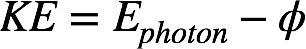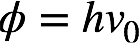# Problem: What is the threshold frequency for sodium metal if a photon with frequency 6.66 × 1014 s−1 ejects an electron with 7.74 × 10 −20 J kinetic energy? Will the photoelectric effect be observed if sodium is exposed to orange light?

🤓 Based on our data, we think this question is relevant for Professor Berrie's class at KU.

###### FREE Expert Solution

Recall: According to the photoelectric effect, an electron can be ejected from the surface of a metal if it's hit with photons of sufficient energy. The kinetic energy (KE) of the ejected electron is related to the energy of the photon (Ephoton) hitting the metal surface by this equation:

(1)where φ = work function of the metal, which is the minimum energy of a photon that is needed to eject an electron. The threshold frequency, v0, of a metal is the minimum frequency needed to eject an electron and is given by:

(2)where h = Planck's constant (6.626 × 10–34 J • s).###### Problem Details

What is the threshold frequency for sodium metal if a photon with frequency 6.66 × 1014 s−1 ejects an electron with 7.74 × 10 −20 J kinetic energy? Will the photoelectric effect be observed if sodium is exposed to orange light?